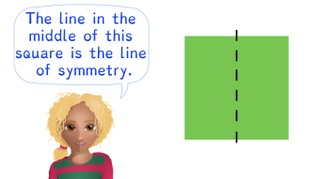Recognizing lines of symmetry

# Recognizing lines of symmetry8,000 schools use Gynzy92,000 teachers use Gynzy1,600,000 students use Gynzy

## General

Students learn to recognize lines of symmetry in shapes and images.

## Standards

CCSS.Math.Content.-4.G.A.3

## Learning objective

Students will be able to recognize lines of symmetry within shapes.

## Introduction

Show a selection of shapes on the interactive whiteboard and ask students which of them have symmetry. Then ask students which tool they can use to help determine if a shape has symmetry. You can choose to use a mirror on the board to show the symmetry of a shape.

## Instruction

Explain to students that a line of symmetry divides a shape into two identical parts. Tell students that when you place a mirror on a line of symmetry, the figure remains exactly the same. Show an example of a shape in which the line of symmetry is in the correct place. Then show a shape in which the line of symmetry is in the incorrect place. Ask if students can determine where the line of symmetry belongs on the next given shape. Have the check their line of symmetry by using a mirror. Then students must draw different shapes in which they must determine where to draw the line of symmetry. Then explain to students that lines of symmetry are not only horizontal or vertical, but can be angled as well. Sometimes you must look very closely to see if a line of symmetry is correctly placed or not. Particularly with colors, it is important to make sure that the line is in the correct place.

Check that students are able to recognize lines of symmetry by asking the following questions:
- What is a line of symmetry?
- What must you pay attention to when determining where the line of symmetry belongs?

## Quiz

Students are given exercises in which they must determine if the line of symmetry is placed correctly or incorrectly. They must also select which of the given images has a correctly placed line of symmetry.

## Closing

Discuss the importance of recognizing the line of symmetry with students. Show students a few shapes and ask them if the line of symmetry is placed correctly or incorrectly. If they believe it is placed correctly, they should stand up. If it is incorrect, they should stay seated. Then ask students to grab three objects that are yellow, red, and blue (for example colored pencils). Show them the picture and ask them to hold up the object that has the same color as the correct line of symmetry.

## Teaching tips

Students who have difficulty recognizing lines of symmetry can be supported by making use of a mirror. Once they are more comfortable with working with the mirror, they should be able to start recognizing where the line of symmetry belongs without the mirror.

## Instruction materials

mirrors, paper, pencils, and objects that are yellow, red, and blue.

### The online teaching platform for interactive whiteboards and displays in schools

• Save time building lessons

• Manage the classroom more efficiently

• Increase student engagement